# Ice and water

We want to cover rectangular rink with dimensions of 55 m and 25 m with 4cm thick layer of ice. How many liters of water we need if after freezing water increases its volume by 10%?

V =  50000 l

### Step-by-step explanation:Did you find an error or inaccuracy? Feel free to write us. Thank you!Tips to related online calculators
Do you know the volume and unit volume, and want to convert volume units?
Tip: Our Density units converter will help you with the conversion of density units.

#### You need to know the following knowledge to solve this word math problem:

We encourage you to watch this tutorial video on this math problem:

## Related math problems and questions:

• IcerinkRectangular rink with dimensions of 68.7 m and 561 dm must be covered with a layer of ice 4.2 cm thick. How many liters of water is necessary for the formation of ice when the volume of ice is 9.7% greater than the volume of water.
• FreezerThe freezer has the shape of a cuboid with internal dimensions of 12 cm, 10 cm, 30 cm. A layer of ice of 23 mm thick was formed on the inner walls (and on the opening) of the freezer. How many liters of water will drain if we dispose the freezer?
• The bodyThe body has dimensions of 2m, 2dm, and 10 cm. It weighs 28 kg. What is its density?
• Water level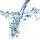To cuboid-shaped poll, bottom size 2m and 3.5m; flows water at a rate of 50 liters per minute. How long will it take for water to reach level 50 cm?
• Cuboid 5Calculate the mass of the cuboid with dimensions of 12 cm; 0.8 dm and 100 mm made from spruce wood (density = 550 kg/m3).
• The cuboidThe cuboid is full to the brim with water. The external dimensions are 95 cm, 120 cm, and 60 cm. The thickness of all walls and the bottom is 5 cm. How many liters of water fit into the cuboid?
• RainfallOn Thursday, fell 1 cm rainfall. How many liters of water fell to rectangular garden with dimensions of 22 m x 35 m?
• IcebergWhat is the surface area of 50 cm iceberg (in the shape of a cuboid) that can carry a man with luggage with a total weight of 120 kg?
• Water tankWhat is the height of the cuboid-shaped tank with the bottom dimensions of 80 cm and 50 cm if the 480 liters of water reach 10 cm below the top?
• AquariumThe box-shaped aquarium is 40 cm high; the bottom has dimensions of 70 cm and 50 cm. Simon wanted to create an exciting environment for the fish, so he fixed three pillars to the bottom. They all have the shape of a cuboid with a square base. The base edg
• Unknown metal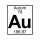The prism made of unknown metal has dimensions of 3 cm by 3 cm by 5 cm and a weight of 121 g. What metal is it? Write its chemical symbol.
• Water levelHow high is the water in the swimming pool with dimensions of 37m in length and 15m in width, if an inlet valve is opened for 10 hours flowing 12 liters of water per second?
• The glass1 m3 of glass weighs 2600 kg. Calculate the weight of the glass glazing panel with dimensions of 2.5 m and 3.8 m if the thickness of the glass is 0.8 cm
• Wood cuboidWhat is the weight of the wood cuboid 15 cm, 20 cm, 3 m if 1 m3 wood weighs 800 kg?
• Stone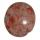When Peter threw stone in a box of water he discovered that the water level has risen by 6 cm. The box has a cuboid shape, the bottom has dimensions of 24 cm and 14 cm, height is 40 cm. What volume has a stone?
• Water overflow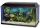A rectangular container that has a length of 30 cm, a width of 20 cm, and a height of 24 cm is filled with water to a depth of 15 cm. When an additional 6.5 liters of water are poured into the container, some water overflows. How many liters of water over
• Snails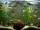How many liters of water will fit in an aquarium with bottom dimensions of 30 cm and 25 cm and a height of 60 cm, if we pour water up to a height of 58 cm? How many most snails can we keep in an aquarium, if we know that snails need 600 cm ^ 3 of water fo